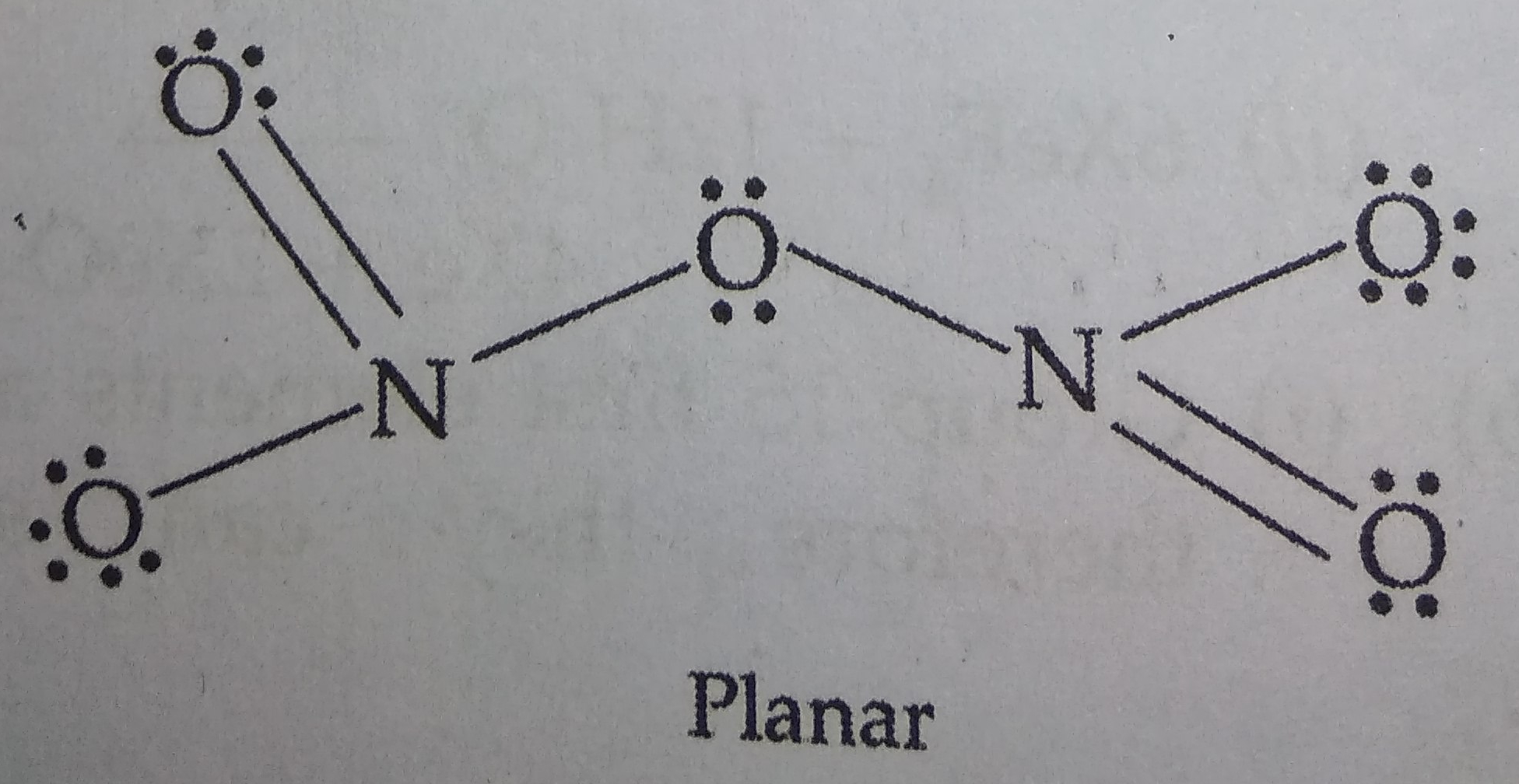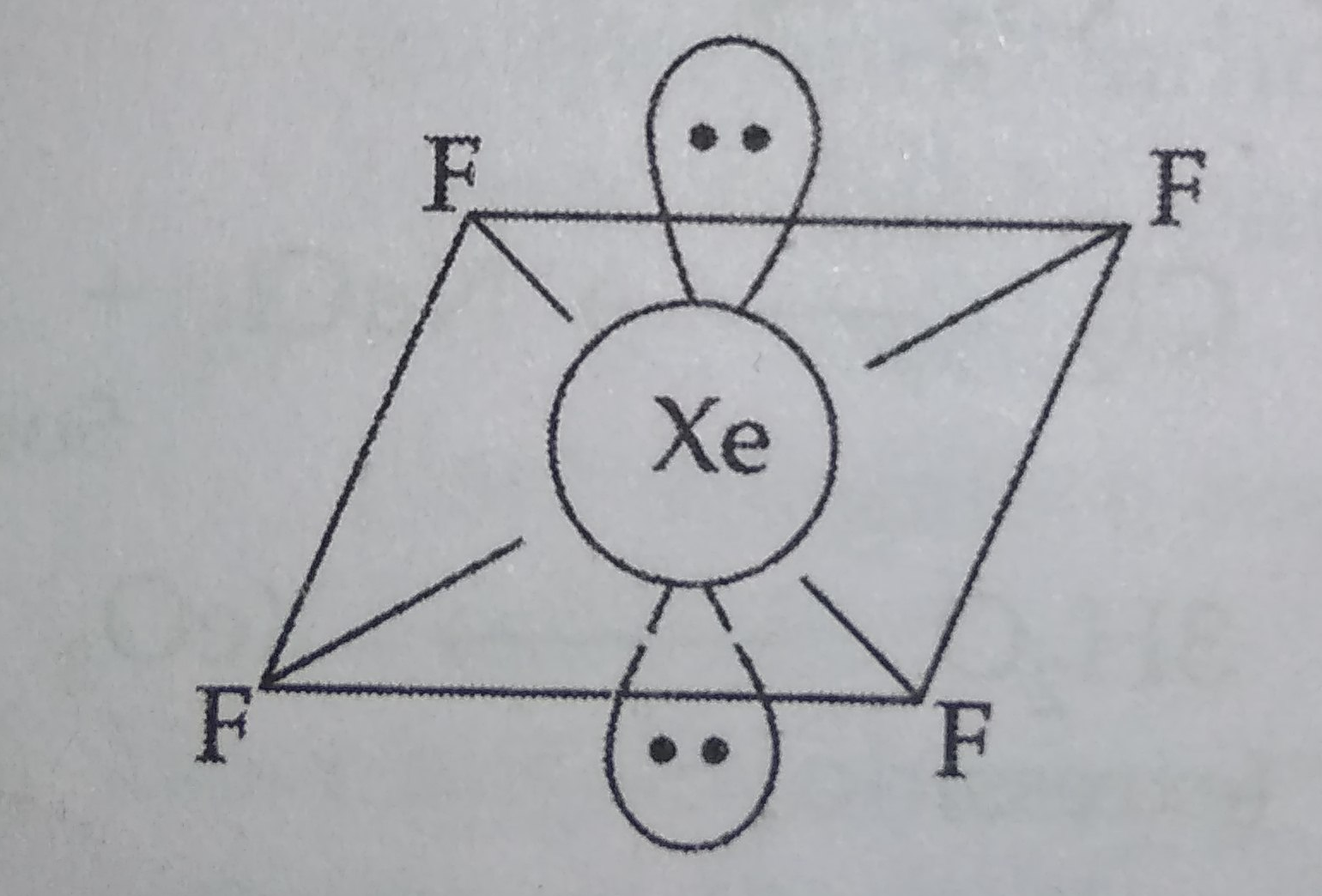(a) Draw the structures of the following :

(i) ${N}_{2}{O}_{5}$                               (ii) $XeO{F}_{4}$

(b) Explain the following observations :

(i) The electron gain enthalpy of sulphur atom has a greater negative value than that of oxygen atom.

(ii) Nitrogen does not form pentahalides.

(iii) In aqueous solutions HI is a stronger acid than HCl.

(a) (i) ${N}_{2}{O}_{5}$(ii) $XeO{F}_{4}$Shape : Square pyramidal

(b) (i) Because enthalpy of dissociation of S-S bond is higher than O-O bond and the hydration energy of ${S}^{2-}$ is less than that of ${O}^{2-}$ ion.

(ii) Due to absence of empty d-orbitals, ${N}_{2}$ does not form pentahalides.

(iii) Due to lower bond dissociation energy and higher degree of ionization, HI acts as stronger acid than HCl in aqueous solution.

Difficulty Level: